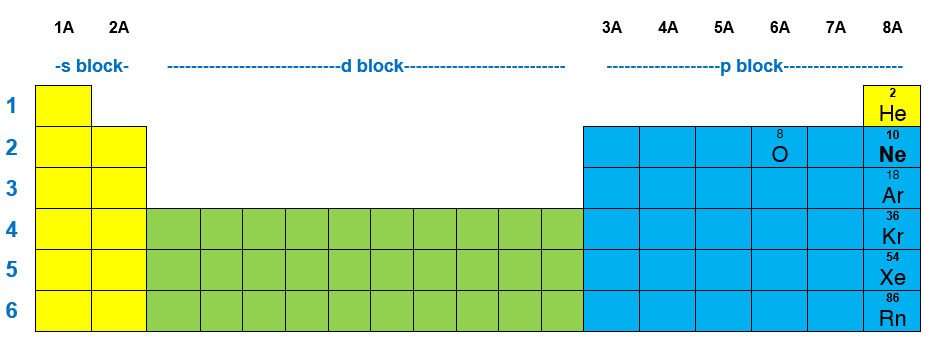# Problem: For an atom of oxygen, what is the greatest number of hybridized orbitals it can have?A. 1 B. 3 C. 6 D. 4 E. 0

###### FREE Expert Solution

Determine the electron orbitals of the valence electrons of an Oxygen atom and figure out how many orbitals can form hybrids

Recall that for a neutral element, Atomic number = # of protons = # of electrons.

The atomic number of O is 8 which means O has 8 electrons. The location of O in the periodic table is shown below:92% (277 ratings)###### Problem Details

For an atom of oxygen, what is the greatest number of hybridized orbitals it can have?

A. 1

B. 3

C. 6

D. 4

E. 0